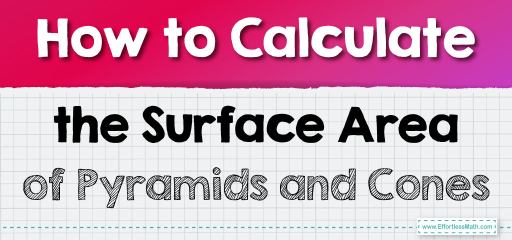# How to Calculate the Surface Area of Pyramids and Cones

The mysteries of the ancient pyramids and the delicious appeal of an ice cream cone have one thing in common - their distinctive shapes! Beyond their allure and history, pyramids and cones are geometric wonders. How much space does their surface cover? What mathematical intricacies lie behind their slanted sides? In this comprehensive guide, we will uncover the techniques to calculate the surface area of pyramids and cones, making these enigmatic shapes more accessible. Let's embark on this intriguing journey through the slopes of geometry!## Step-by-step Guide: Surface Area of Pyramids and Cones

Pyramid:

The surface area  of a pyramid can be found using the formula: Surface Area $$SA = \text{Base Area} + \frac{1}{2} \times \text{Perimeter of Base} \times \text{Slant Height}$$

Cone:

The surface area  of a cone can be found using the formula: Surface Area $$SA = \pi r^2 + \pi r \times \text{Slant Height}$$

Where:
$$r =$$ radius of the base

### Examples

Example 1:
Find the surface area of a square pyramid with a base side of $$5 \text{ cm}$$ and a slant height of $$7 \text{ cm}$$.

Solution:
Base Area $$= 5 \text{ cm} \times 5 \text{ cm} = 25 \text{ cm}^2$$

Perimeter of Base $$= 4 \times 5 \text{ cm} = 20 \text{ cm}$$

$$SA = 25 \text{ cm}^2 + \frac{1}{2} \times 20 \text{ cm} \times 7 \text{ cm} = 95 \text{ cm}^2$$

Example 2:
Calculate the surface area of a cone with a base radius of $$6 \text{ cm}$$ and a slant height of $$10 \text{ cm}$$.

Solution:
$$SA = \pi (6 \text{ cm})^2 + \pi (6 \text{ cm})(10 \text{ cm}) = 301.44 \text{ cm}^2$$

### Practice Questions:

1. Determine the surface area of a triangular pyramid with a triangular base of area $$15 \text{ cm}^2$$, base perimeter of $$18 \text{ cm}$$, and a slant height of $$9 \text{ cm}$$.
2. What is the surface area of a cone with a radius of $$4 \text{ cm}$$ and a slant height of $$8 \text{ cm}$$?

1. $$96 \text{ cm}^2$$
2. $$150.8 \text{ cm}^2$$

### What people say about "How to Calculate the Surface Area of Pyramids and Cones - Effortless Math: We Help Students Learn to LOVE Mathematics"?

No one replied yet.

X
30% OFF

Limited time only!

Save Over 30%

SAVE $5 It was$16.99 now it is \$11.99